Miscellaneous

Chapter 6 Class 12 Application of Derivatives
Serial order wise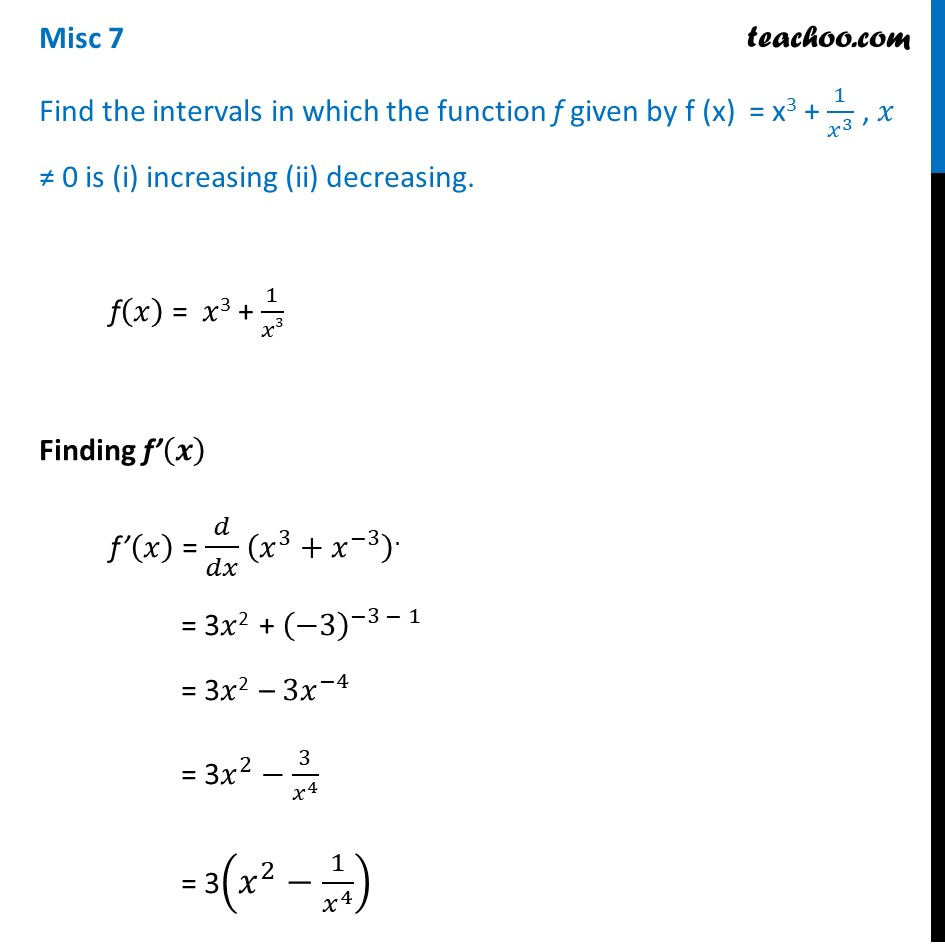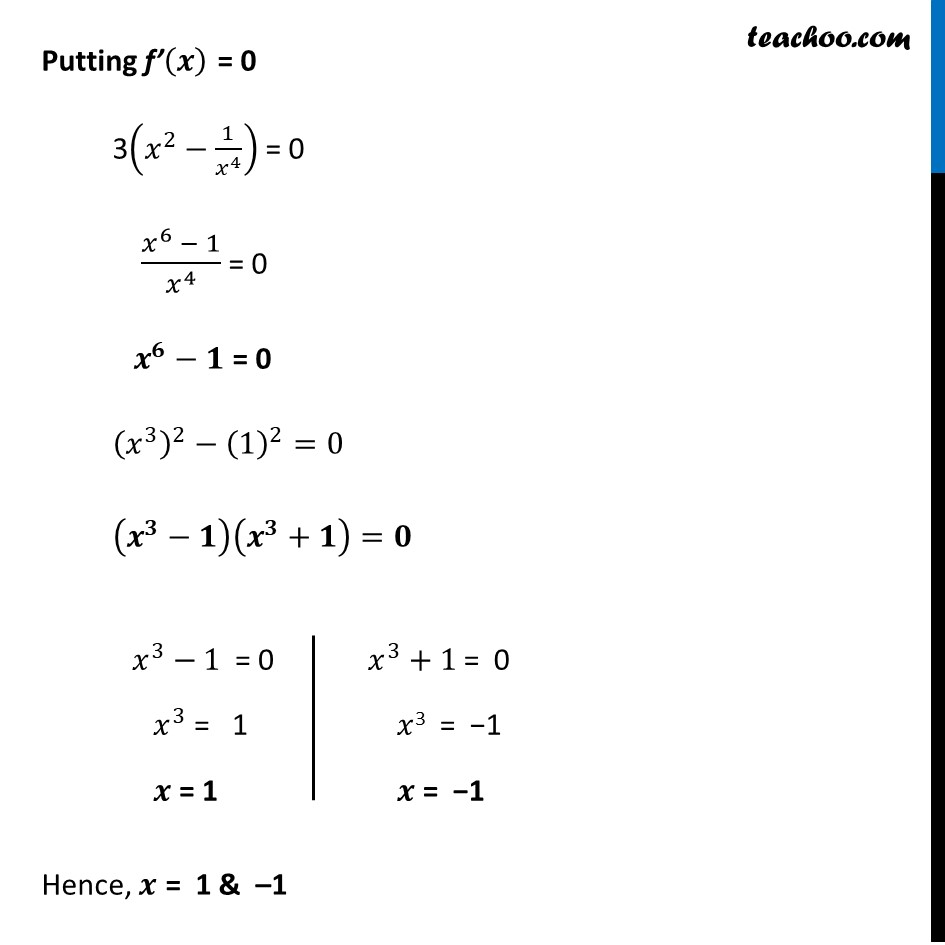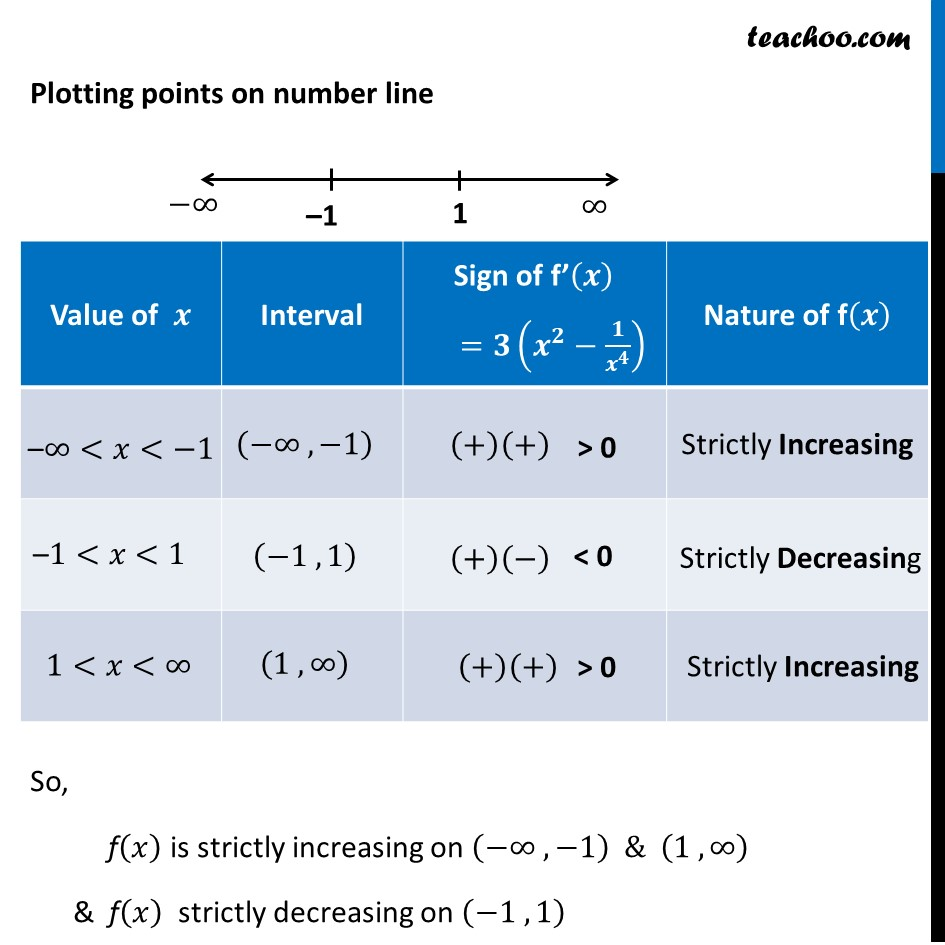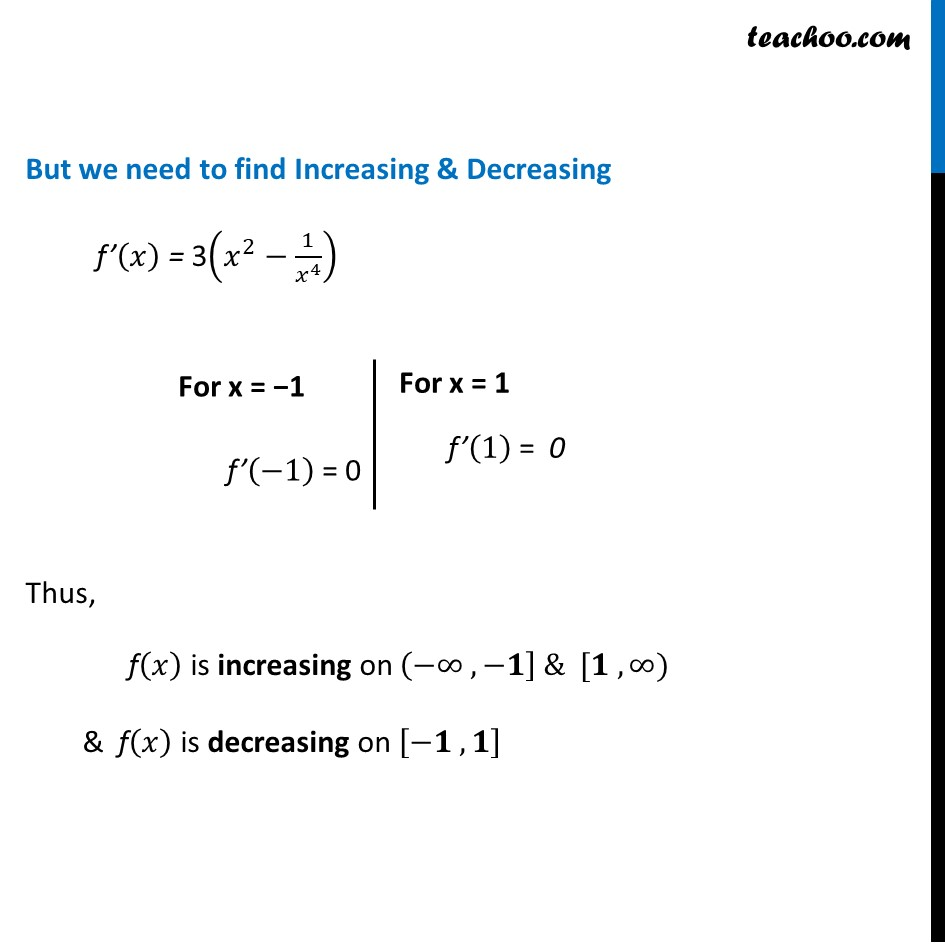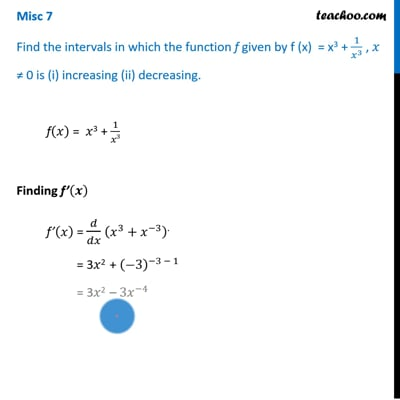This video is only available for Teachoo black users

Solve all your doubts with Teachoo Black (new monthly pack available now!)

### Transcript

Misc 7 Find the intervals in which the function f given by f (x) = x3 + 1/𝑥^3 , 𝑥 ≠ 0 is (i) increasing (ii) decreasing. f(𝑥) = 𝑥3 + 1/𝑥3 Finding f’(𝒙) f’(𝑥) = 𝑑/𝑑𝑥 (𝑥^3+𝑥^(−3) )^. = 3𝑥2 + (−3)^(−3 − 1) = 3𝑥2 – 3𝑥^(−4) = 3𝑥^2−3/𝑥^4 = 3(𝑥^2−1/𝑥^4 ) Putting f’(𝒙) = 0 3(𝑥^2−1/𝑥^4 ) = 0 (𝑥^6 − 1)/𝑥^4 = 0 𝒙^𝟔−𝟏 = 0 (𝑥^3 )^2−(1)^2=0 (𝒙^𝟑−𝟏)(𝒙^𝟑+𝟏)=𝟎 Hence, 𝒙 = 1 & –1 𝑥^3+1 = 0 𝑥3 = −1 𝒙 = −1 Plotting points on number line So, f(𝑥) is strictly increasing on (−∞ , −1) & (1 , ∞) & f(𝑥) strictly decreasing on (−1 , 1) But we need to find Increasing & Decreasing f’(𝑥) = 3(𝑥^2−1/𝑥^4 ) Thus, f(𝑥) is increasing on (−∞ , −𝟏] & [𝟏 , ∞) & f(𝑥) is decreasing on [−𝟏 , 𝟏] For x = −1 f’(−1) = 0 For x = 1 f’(1) = 0